### Question 13. Solve the following example. A ball falls off a table and reaches the ground in 1 s. Assuming g = 10 m/s2, calculate its speed on reaching the ground and the height of the table.

Question 13.

Solve the following example.

A ball falls off a table and reaches the ground in 1 s. Assuming g = 10 m/s2, calculate its speed on reaching the ground and the height of the table.

From the Newton’s first law of motion;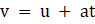V = Final velocity;

U = initial velocity;

T = time taken;

A = acceleration;

According to our question;

u = 0 (ball is at table and is falling from it (free fall) hence its initial velocity is zero);

A = 10m/s2 (because when object will be down the acceleration due to gravity will be acting downwards to make object to fall. Hence by sign convention direction of motion and acceleration is same therefore a is positive);
T = 1 sec ( given);

The figure below describes the ball falling from the table on ground with final velocity v.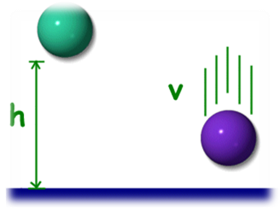Putting the values we get;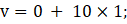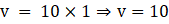Hence final velocity on reaching the ground is 10m/s.

Height of table Now, By Newton’s second equation of motion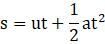According to our question;

s = Table height;

u = 0 (ball is at table (maximum height) and is falling from it hence its initial velocity is zero);

a = 10m/s2.

t = 1s.

Putting the above values we get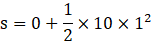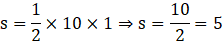Hence height of the table is 5m